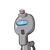# In fig, triangle ABC is drawn circumscribing a circle of radius 3 cm, such that segments BD and DC into which BC is divided by the

In fig, triangle ABC is drawn circumscribing a circle of radius 3 cm, such that segments BD and DC into which BC is divided by the point of contact D and are of lengths 6 cm and 8 cm respectively. Find side AB if the area of triangle ABC is 63 cm^2​

### 1 thought on “In fig, triangle ABC is drawn circumscribing a circle of radius 3 cm, such that segments BD and DC into which BC is divided by the”

1.